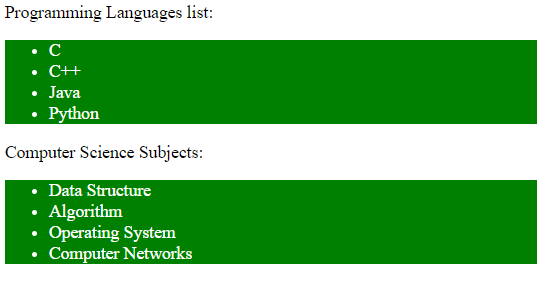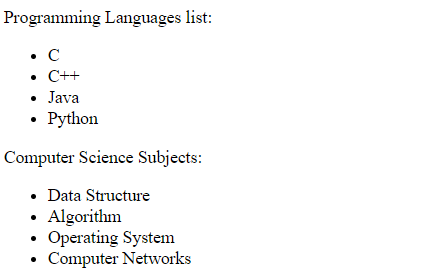# CSS element1~element2 Selector

The element1~element2 selector in CSS is used to match the occurrences of element2 followed by element1. It is not important to element1 immediately followed by element2. This property works if both elements have a common parent element.

Syntax:

```element1~element2 {
/* CSS Property */
}```

Example 1: In this example, both elements have the same parent element (body).

## html

 `` `<``html``>`   `<``head``>` `    ``<``title``>` `        ``CSS element1~element2 Selector` `    ```   `    ``<``style``>` `        ``p~ul {` `            ``background: green;` `            ``color: white;` `        ``}` `    ``` ``   `<``body``>` `    ``<``div``>` `        ``<``p``>Programming Languages list:` `        ``<``ul``>` `            ``<``li``>C` `            ``<``li``>C++` `            ``<``li``>Java` `            ``<``li``>Python` `        ```   `        ``<``div``>Computer Science Subjects:` `        ``<``ul``>` `            ``<``li``>Data Structure` `            ``<``li``>Algorithm` `            ``<``li``>Operating System` `            ``<``li``>Computer Networks` `        ``` `    ``` ``   ``

Output:Example 2: In this example, both elements do not have the same parent element. So CSS property will not apply.

## html

 `` `<``html``>`   `<``head``>` `    ``<``title``>` `        ``CSS element1~element2 Selector` `    ```   `    ``<``style``>` `        ``p~ul {` `            ``background: lightgrey;` `            ``border: 2px solid green;` `        ``}` `    ``` ``   `<``body``>` `    ``<``p``>Programming Languages list:` `    ``<``div``>` `        ``<``ul``>` `            ``<``li``>C` `            ``<``li``>C++` `            ``<``li``>Java` `            ``<``li``>Python` `        ```   `        ``<``div``>Computer Science Subjects:` `        ``<``ul``>` `            ``<``li``>Data Structure` `            ``<``li``>Algorithm` `            ``<``li``>Operating System` `            ``<``li``>Computer Networks` `        ``` `    ``` ``   ``

Output:Supported Browsers: The browser supported by element1~element2 Selector are listed below: